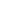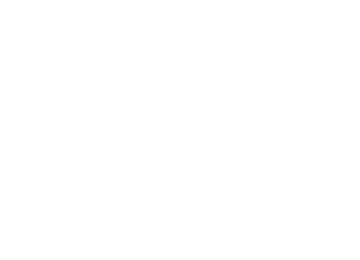### Mock Test 1###### Held on May 9, 2021
3:30 AM###### 75 Q’s · 300 marks
180 mins###### Languages
English#### Syllabus

##### Mathematics
• Circles
• Probability
• Set Theory
• Trigonometric Identities & Equations
• Binomial Theorem
• Matrices & Determinants
• Inequalities and Absolute Value
• Straight Lines
• Functions & Graphs

##### Chemistry
• General Topics & Mole Concept
• Chemical Bonding
• Basic Principle of Organic
• Stereochemistry & Isomerism
• Gases & Liquid States
• Redox Reactions
• Alkanes & Alkyl Halides
• Chemical Kinetics

##### Physics
• Geometrical Optics
• Sound Waves
• Work, Energy & Power
• Kinetic Theory of Gases
• Laws of Motion & Friction
• Surface Tension & Energy
• Centre of Mass & Linear Momentum
• Kinematics
• Basic Mathematics
• Simple Harmonic Motion